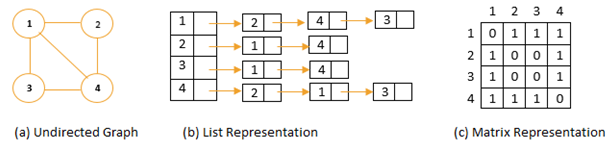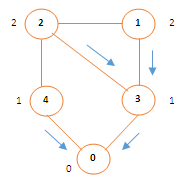# Implementation and Analysis of Beadth First Search(BFS) and Graphs

 Category: Data Structure And Algorithms Tags:Breadth First Search Code Files

## Introduction

In this article we are going to learn about searching a graph. Searching a graph means following edges of graph and find every vertex. Usually we represent a graph as G = (V, E) which is collection of vertices and edges. Breadth-First Search(BFS) is simple search algorithm even though Dijkstra’s single source shortest path is based on same idea.

Before going for implementation we should know how do we represent graphs, we have two approaches to represent graphs are Adjacency List and Adjacency Matrix representation.As we can see above graph representation that is undirected graph, in adjacency representation we maintain links between nodes like Vertex 1 is linked to vertex 2, 3, 4 so we can say edges will leave vertex 1 are (1, 2), (1, 3) and (1, 4). In Matrix representation we simply maintain binary value 0 and 1, if 1 and two are connected we have to mark position (1, 2) as 1 else 0. Matrix representation required memory Θ(V2) but it is easy to understand.

## Implementation

Given a graph G = (V, E) and a source vertex s, breadth-first search will follow all the edges and find new vertices linked to s and from those vertices find other vertices and so on until all vertices are explored. It calculates distance from source s to each reachable vertex. For any reachable vertex v from s shortest path can be calculated. To keep track which vertex has been already found or in progress data structure keeps color attribute which can be white, gray or black. When we start we mark all vertices white then they become gray and in last black. To implement we first will create our vertex data structure:

```/// <summary>
/// Vertex Structure
/// </summary>
public class V
{
public int Vertex { get; set; }  //vertex number
public V Next { get; set; }  //reachable vertex
public V(int val)
{
Vertex = val;
Distance = Int32.MaxValue;
Color = 'W';
}

public int Distance { get; set; } //distance from source
public char Color { get; set; }    //initial color
public V Parent { get; set; }  //parent node for keep tracking direction
}
```

Above code is self-explanatory now we have to build our adjacency list as well perform BFS, for that we will create a class:

```public class BreadthFirstSearch
{
public int v;
public V[] adjList;
public BreadthFirstSearch(int totalVertix)
{
v = totalVertix;
adjList = new V[totalVertix];
for (int i = 0; i < adjList.Length; i++)
adjList[i] = new V(i);

}

public void AddV(int u, int v){...}
private void BFS(int source){...}
public void PrintPath(int u, int v){...}
private void RecPrint(V u, V v){...}
}
```

Above class BreadthFirstSearch is taking number of vertices in constructor and we can are initializing adjacency list of given length. First method is AddV which adds a vertex in adjacency list will be:

```/// <summary>
/// Adds a vertex to adjacency list
/// </summary>
/// <param name="u">vertex where v has to be linked</param>
/// <param name="v">vertex which has to be linked</param>
public void AddV(int u, int v)
{
V tempU = adjList[u];
//finding if there is already same vertex v is connected
while (tempU.Next != null)
{
if (tempU.Vertex != v) //reaching last
tempU = tempU.Next;
else             //v is already defined
return;
}
tempU.Next = new V(v);   // connecting new vertex v to u

//for undirected graph we have to connect vertex v also to u
V tempV = adjList[v];
while (tempV.Next != null)
tempV = tempV.Next;

tempV.Next = new V(u);  //connecting v to u
}
```

Above method adds or links a new vertex, if vertex already exists method will ignore adding it, you can understand the method followed by comments. Now we will see implementation of method BFS which does what exactly we are trying to achieve:

```/// <summary>
/// Breadth First Search Algorithm
/// </summary>
/// <param name="source">where to start</param>
private void BFS(int source)
{
Queue<V> queue = new Queue<V>(); //initializing vertex Queue
V src = adjList[source]; //finding source
src.Color = 'G'; //giving color grey to source
src.Distance = 0; //0 distance to source
src.Parent = null;  // np parent for source

//marking all other vertices color to white distance is max and parent is null
for (int i = 0; i < adjList.Length; i++)
{
V u = adjList[i];
if (u.Vertex != source)
{
u.Color = 'W';
u.Distance = Int32.MaxValue;
u.Parent = null;
}
}

queue.Enqueue(src); //enquing source
while (queue.Count > 0)
{
V u = queue.Dequeue();
V v = u.Next; //finding linked vertex
while (v != null)
{
V mainV = adjList[v.Vertex];  // getting actual vertex
if (mainV.Color == 'W')  //process only if white
{
mainV.Color = 'G'; //grey for currently processing
mainV.Distance = u.Distance + 1; // distance 1+ from parent
mainV.Parent = u; //assigning parent
queue.Enqueue(mainV); //enqueue for finding connected nodes to this
}

v = v.Next; //another node connected to u
}

u.Color = 'B'; //once completed mark color as black
}
}
```

Above method maintains a queue to explore all vertices, first it marks all vertices white and source as grey, actually white is for vertex which are not yet explored, grey is for explored but still being processed and black is for processed ones. After this we enqueue the source and find all connected vertices to it then we process them and give two important properties distance and parent and the same will be repeated until all are processed, now let's print path:

```/// <summary>
/// Recursive method prints minimum path
/// </summary>
/// <param name="u">source</param>
/// <param name="v">destination</param>
private void RecPrint(V u, V v)
{
if (u == v)
Console.WriteLine(u.Vertex);
else if (v.Parent == null)
Console.WriteLine("No way from u to v");
else
{
RecPrint(u, v.Parent);
Console.WriteLine(v.Vertex + " ");
}
}
```

Above method prints the correct shortest path between source and distance now we call these methods from our public method:

```public void PrintPath(int u, int v)
{
BFS(u);
RecPrint(adjList[u], adjList[v]);
}
```

Now we will execute this code:

```static void Main(string[] args){    BreadthFirstSearch demo = new BreadthFirstSearch(5);    demo.AddV(1, 3);    demo.AddV(2, 4);    demo.AddV(2, 1);    demo.AddV(0, 3);    demo.AddV(3, 1);    demo.AddV(4, 0);    demo.AddV(3, 2);    demo.PrintPath(0, 1);    Console.ReadLine();}
```

Output

0

3

1

Above we are adding vertices which will form adjacency list:

0 → 3 → 4

1 → 3 → 2

2 → 4 → 1 → 3

3 → 1 → 2 → 0

4 → 0 → 2

And after execution of PrintPath method graph will be marked as:Inside circle is Vertex and outside one is Distance from source, if we want path from Vertex1 to Vertex0, Distance will be 2 and program will print path: 1 3 0

## Analysis

We can see above that one vertex will be explored only once because of color so there complexity is O(V) and we are exploring all adjacency list also which is actually edges so that complexity will be O(E) so total complexity of BFS will be O(V+E).

 Like0 People
Last modified on 31 October 2018Nikhil Joshi Ceo & Founder at Dotnetlovers Atricles: 144 Questions: 15 Given Best Solutions: 15 *

## Comments:

No Comments Yet

You are not loggedin, please login or signup to add comments:

## Existing User

Login via:## New Userx